# Is impulse change in momentum?

From Newton’s second law of motion, we know that if a force is applied to a body then the body accelerates in the direction of the applied force.

By definition, acceleration is the rate of change of velocity, in the form of an equation this is represented as: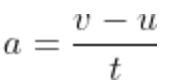Therefore, F = ma can be written as: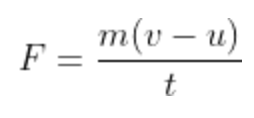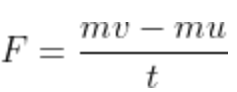Since (mv – mu) is the change of momentum, therefore,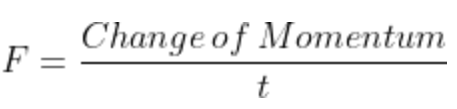If a force F is applied on a body of mass m for a time interval Δt and if the change in velocity is Δv then impulse is given as follows: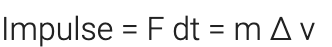We know that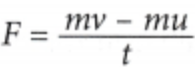Rearranging the equation, we get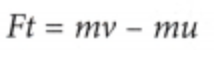The quantity Force × Time is called the impulse.(6)(0)